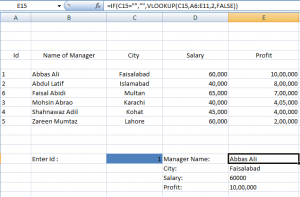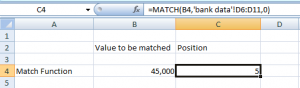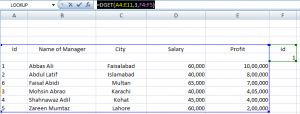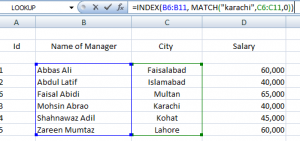# 6 Ways to Search Data in Excel

Microsoft Excel is used to create spreadsheets that contain massive amounts of data. It could be a thousand rows and a twenty-five column data or more than that. With such big data, it becomes very difficult to find a particular record on basis of some criteria. All the accountants could have scratched their heads off if Microsoft Excel had not provided them with the built in functions. It contains several search functions that help you find a particular record or its position from your data. We will look into all these functions one by one.

## LookUp Function:

It looks up a value either from a one-row or one-column range or from an array. You can search for a particular value by using the function:

=Lookup(Lookup_value, array)

Where, array defines the range of column or a row from which you want to retrieve a value.

## HLookUp Function:

HLookup Function is a broader approach of the basic lookup function. It finds a value in the top row of a table or array of values and returns the value in the same column from a row you specify. It has the syntax:

=HLookUp(lookup_value, table_array, row_index_num, range_lookup)

Where,

• Lookup value is the first value of the column.
• Table array describe the range of cells covering data set.
• Row index number is the row number from which you want to return the result.
• False means that the HLookUp function won’t search for the exact match.

Consider the following example,

=HLOOKUP(B6,A6:E11,3,FALSE)

With this formula, we are extracting the value of 3rd row in the B column which starts from B6. A6:E11 contains our complete data.

## VLookUp Function:

VLookup function is very commonly used for the search purposes. It searches the column from left to right but can not reverse its direction. It helps you to lookup a specific value in the left most columns, and then it will return a value from the same row from the column you specify. For example, in our data set we enter the ID and we can retrieve any attribute of that employee like name, city or salary. The formula is similar to HLookUp. However, we do not mention the top value of any column here. It has four arguments:

• lookup_value: the value to be searched
• table_array: data set on which you want to perform search.
• col_index_num: the index of your column. For example, ‘Name of manager’ has index 2.
• range_lookup: it can be true or false. True means that the function searches for an exact match of the value.

You can make a simple widget using the VLookUp function which takes the ID as input and displays all information of that person. We have used ‘If’ function which checks that the widget does not display any result if the ID cell is empty. All the attributes Manager Name, City, Salary and Profit are using the same formula (as the one displayed in the formula bar) with a difference in Column Index. It is 2 for manager name, 3 for city, 4 for salary and 5 for the profit; the rest remains the same.## Index Function:

Index function returns a value of the cell at the intersection of particular row and column. It gives you a specific item/value from a specific position and a specific column. For example, you can find what the sixth item is in the second column with the help of index.

It has easy to understand syntax: Index (array, row_num, column_num)

Where,

• Array is the range of cells on which you will perform the search. It could be your entire data set.
• Row_num and Column_num are the row number and the column number from which the value would be returned.

We applied the Index function in a separate worksheet and our data sheet is entitled as ‘bank data’. We wanted to find the third element in the eighth row.

1-      Select the cell and type “=INDEX()”.

2-      The first argument is your entire data set which is A6:E11 in our case.

3-      The eighth row occupied the cell range A8 to E8.

4-      The last argument is the column number.

5-      Note that ‘bank data’ is the name of sheet from where we are extracting information. If we are using a single sheet to maintain the data and use Index function, we will give the cell references only.

6-      Your formula will resemble this:

=INDEX(‘bank data’!A6:E11,’bank data’!A8:E8,3)

7-      Press Enter and see the result.

## Match Function:

It returns the position of a value that matches a specified value. However, if you have several occurrences of the look up value, then it will return the position of first occurrence only. It has three arguments:

• Lookup_value: this is the value that will be matched against existing record. You may give a constant here or cell reference that contains the value.
• Lookup_array: It is the column which will contain the look up values.
• Match_type: It describes whether you want an exact match, greater or lesser than look up value represented by numbers 0, 1 and -1 respectively.

The procedure of using the formula is the same as that of Index function. Now, you may easily observe that we referred the cell in the look up value parameter. ‘bank data’!D6:D11 is the column range or the look up array. ‘0’ indicates that we need an exact match for the value in B4. Press enter and it returns the position ‘2’ of the value ’40,000’.## DGet Function:

DGet function is categorized in the database functions. It is used to extract a single value from the database on basis of certain criteria. It has three parameters: database, field and criteria.

You will mention the cell range covering database in the first parameter. Field is the column name or number from where you want to retrieve record. Criteria contain two cells (the column name and its value) which will be used to filter the information from entire database.

For example, the formula DGET(A4:E11,3,F4:F5) returned Faislabad as the result.## How to use Functions Together:

As we have seen that VLookUp function works with many limitations. Similarly, we could not derive any important results using the Index or Match functions. You will be amused to know how we can combine two functions of Index and Match to make a more powerful combination. This combination will overcome the limitations of VLookUp function.

Our technique is to return the index of a particular value using the Match formula. This result will be passed into the Index which will retrieve the value from that position. It will give us the following formula:

=Index(array, Match(search value, search column, 0))

Consider the following example, I wanted to know the ‘Name of Manager’ in ‘Karachi City’. Vlookup fails here because it can not go from right to left. Index function needs the exact row position from where the record is to be extracted. So, we used the Match function to give us the row position.

Match(“Karachi”,C6:C11,0)

Where, C6:C11 is the array of city.

It will return the row number of Karachi which is passed as parameter in index. This row number is searched against the particular ‘Name of Manager’ column. So, our final formula becomes:

=Index (B6:B11, Match(“Karachi”,C6:C11,0) )

Where, B6:B11 refers to the Name of Manager column.

Note that that the column_num parameter in Index function is optional.Let’s keep it till here for today. In our upcoming posts, we will discuss the problems that one may come across using these functions and how to overcome them.

Till then, Stay tuned!